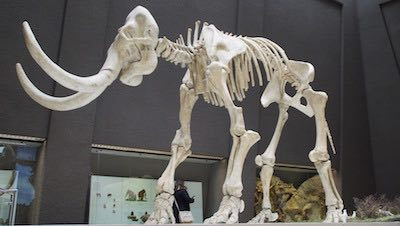### The Number Comma with

Sums# When used in sums, the number comma separates number classes from each other.

Use the Comma with Sums of More than One Number Class

This use of the number comma with sums is also called the counting comma. The counting comma has only one job: it separates number classes of the decimal system from each other. You’re probably familiar with four or five of these number classes (also called number periods). These are the first six:

• Units

• Thousands
• Millions
• Billions
• Trillions

## Numbers

• 1+

• 1,000+
• 1,000,000+
• 1,000,000,000+
• 1,000,000,000,000+
• 1,000,000,000,000,000+

• Units

• Thousands
• Millions
• Billions
• Trillions

## Numbers

• 1+

• 1,000+
• 1,000,000+
• 1,000,000,000+
• 1,000,000,000,000+
• 1,000,000,000,000,000+

You’ll likely never handle numbers higher than the quadrillion class. But even if you do, the concept continues all the way to infinity: use a counting comma to separate number classes from each other.

Numbers in the thousands, for instance, use a counting comma to separate the class of units from the class of thousands:

123,224

Even when you write a number with words instead of numerals, the same rule applies; separate number classes with a counting comma:

One thousand twenty-three,two hundred twenty-four

Notice the one difference in the two examples above, however. When the counting comma is used between numerals, it doesn’t use a space after it. When used with words, however, the counting comma does use a space.

An easy way to remember when to use the counting comma is to memorize that a counting comma is always inserted after every third digit from the left of the decimal point.

p

We use the counting comma simply because it makes large numbers easier to read. Which, for instance, would you rather read:

### 85,392,837

Don’t Use It with Remainders or Numerical Identifiers

The counting comma only appears on the left side of a decimal point (the integer-part of a number). Never use a counting comma in the fractional-part of a number (the right side of a decimal point):

6,134,523.42389

Further, the counting comma only appears in numbers that represent sums. Numbers that work as identification marks do not use counting commas. This includes street addresses, page numbers, numerical codes, and years:

Item number 24394 vanished from the museum in 1994.

Conventionally, however, years with five or more digits do use the counting comma:

19,000 BCEKnowledge Check

R
Q

Choose the option that correctly uses the counting comma.Previous Lesson

Next Lesson